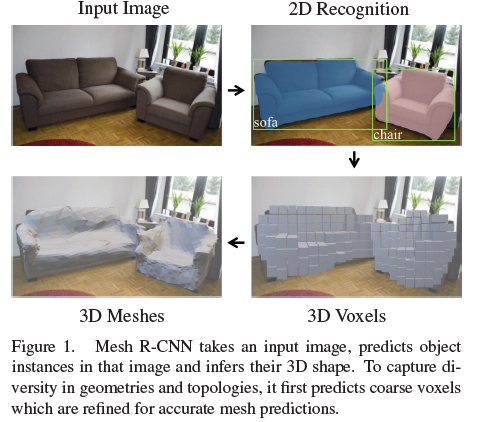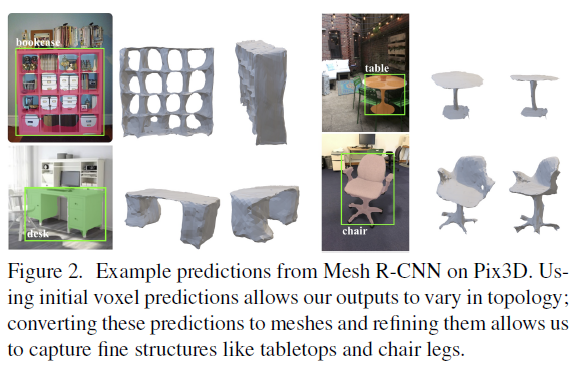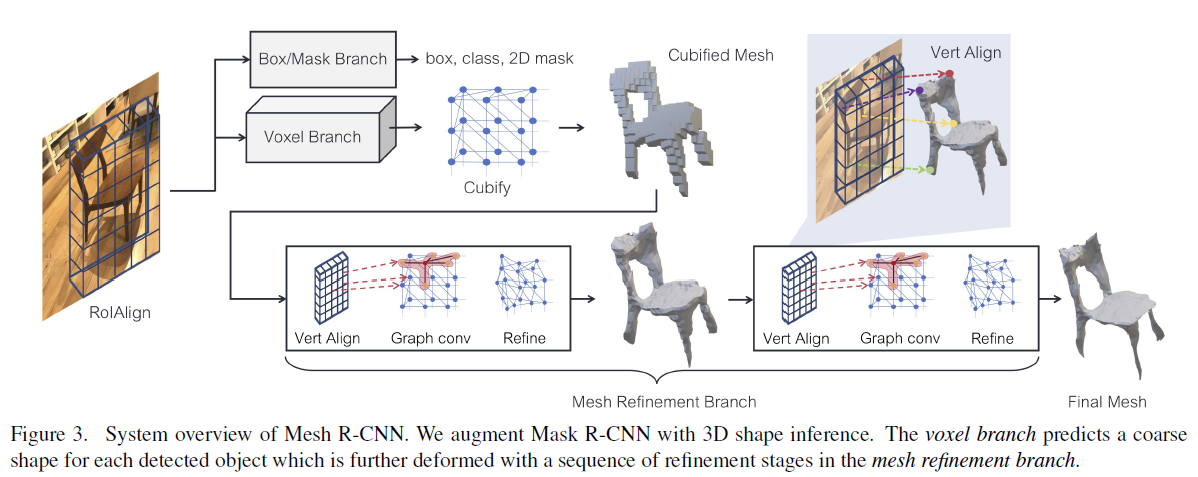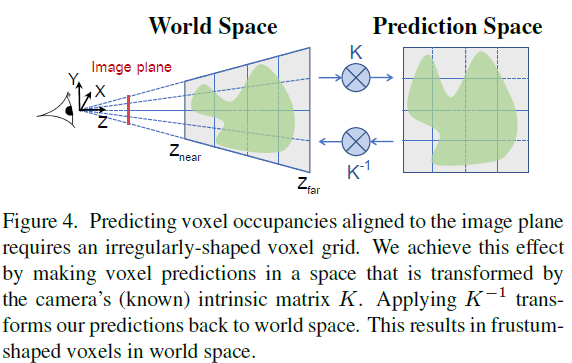# 【Mesh R-CNN】论文翻译（原理部分）

2020/02/04 17:55

Mesh R-CNN 论文翻译（实验部分）见https://www.cnblogs.com/windsing/p/12344936.html

## 1.介绍## 2.相关工作

一些方法预测规则体素网格；然而直观，缩放到高分辨率输出需要复杂的八叉树[49,61]或嵌套的形状架构。

其他的直接输出三角形网格，但被限制从固定的[55,56,68]或检索的网格模板变形，限制了他们可以表示的拓扑。

我们的方法使用了体素预测和网格变形的混合，使高分辨率的输出形状可以灵活地表示任意拓扑。

一些方法在没有3D标注的情况下重建3D图形[23, 25, 47, 67, 74]。这是一个重要的研究方向，但目前我们只考虑完全监督的情况，因为我们成功地对二维感知进行了强有力的监督。

3D输入　我们的方法输入二维图像，预测语义标签和三维形状。由于深度传感器的可用性越来越高，人们对从三维输入（RGB-D图像，点云）中预测语义标签的方法越来越感兴趣。我们预计，将3D输入纳入我们的方法可以提高形状预测的保真度(fidelity)。

## 3.方法我们现在详细描述我们的网格预测器，包括体素分支和网格细化分支，以及它们的相关损失。

### 3.1网格预测

#### 3.1.1体素分支

与Mask R-CNN一样，我们通过在RoIAlign生成的输入特征图上应用一个小的全卷积网络来维持输入特征与预测体素之间的对应关系。该网络生成一个带有$G$通道的特征图，为输入中的每个位置提供一个体素占用分数列。

在3D中，保持图像和我们的预测之间的像素对应是很复杂的，因为当物体远离时，它们会变得更小。如图4所示，我们通过使用相机的(已知的)固有矩阵来预测体素。（预测体素在接收面的位置需要一个不规则形状的体素网格。

CuBify: Voxel to Mesh　体素分支产生一个三维网格的占用概率，给出一个物体的粗糙形状。为了预测更精细的3D形状，我们希望将这些体素预测转换为一个三角形网格，该三角形网格可以传递给网格细分分支（mesh refinement branch）

我们用一种叫做cubify的操作来填补这个空白。它输入体素占用概率和体素占用的二值化阈值。每个被占据的体素被一个长方体三角形网格替换，它有8个顶点，18条边和12个面。合并相邻已占用体素之间的共享顶点和边，并消除共享的内部面。这样就形成了一个水密网格，其拓扑结构依赖于体素预测。

Cubify必须是高效和批量的。这不是小事，我们在附录中提供了实现这一点的技术实现细节。或者，移动立方体（marching cubes）可以从体素网格中提取等值面，但是要复杂得多。

Voxel Loss　体素分支被训练来最小化预测体素占用概率和真实体素占用之间的二元交叉熵。

#### 3.1.2网格细分分支

Mesh Loss　定义在三角形网格上本地操作的损失是具有挑战性的，所以我们使用定义在有限点集上的损失函数。我们通过密集采样点云的表面来表示一个网格。因此，点云损失近似于形状上的损失。与类似，我们使用可微分网格采样从网格表面均匀地采样点(及其法向量)。为此，我们实现了一种高效的批量采样器；详见附录。我们使用这个操作从ground-truth网格中采样点云$P^{gt}$，从我们的模型中每个中间网格预测中采样点云$P^i$。

给定两个点云$P$，$Q$和法向量，令$\Lambda_{P,Q}=\{(p,arg min_q||p-q||):p\in P\}$为一组数据对$(p,q)$集，其中 $q$ 是 $Q$ 中 $p$ 的最近邻居，令 $u_p$ 是点 $p$ 的法向量。点云$P$与$Q$之间的倒角距离为

$L_{cham}(P,Q)=|P|^{-1}\sum\limits_{(p,q)\in \Lambda_{P,Q}}||p-q||^2+|Q|^{-1}\sum\limits_{(q,p)\in \Lambda_{Q,P}}||q-p||^2$

$L_{norm}(P,Q)=-|P|^{-1}\sum\limits_{(p,q)\in \Lambda_{P,Q}}|u_p\cdot u_q|-|Q|^{-1}\sum\limits_{(q,p)\in \Lambda_{Q,P}}|u_q\cdot u_p|$

第i级的网格损失为 $L_{charm}(P^i,P^{gt})$，$L_{norm}(P^i,P^{gt})$，$L_{edge}(V,E)$ 的加权和。网格细分分支被训练来最小化所有细分阶段的平均损失。

0
0 收藏

### 作者的其它热门文章0 评论
0 收藏
0